#  Click-through rate prediction based on feature interaction

## 1. AFM

(1) This paper proposes to add an attention layer on the basis of FM. FM considers the first-order and second-order interactions of features. The FM formula is as follows:Or formulas can be described this way:(2) Based on the above, we take the second-order feature interaction part in FM, and the formula is as follows:(3) The AFM summary structure and formula are as follows: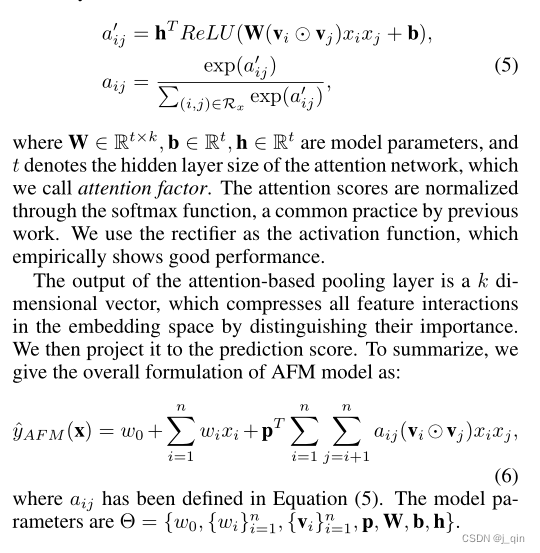(4) The code is as follows:

```def feature_interaction(feature_emb):
p, q = zip(*list(combinations(range(num_fields), 2)))

emb1 = torch.index_select(feature_emb, 1, self.field_p)
emb2 = torch.index_select(feature_emb, 1, self.field_q)
return emb1 * emb2 # [b,(f*f-f)/2,emb]

# attention layer
self. attention = nn. Sequential(nn. Linear(embedding_dim, attention_dim),
nn.ReLU(),
nn.Linear(attention_dim, 1, bias=False),
nn.Softmax(dim=1))

elementwise_product = self. product_layer(feature_emb) # # [b,(f*f-f)/2,emb]
attention_weight = self. attention(elementwise_product) # [b,(f*f-f)/2,1]
attention_sum = torch.sum(attention_weight * elementwise_product, dim=1) # [b,emb]
afm_out = self.weight_line(attention_sum) # After a linear layer, [b,1]
y_pred = self.lr_layer(x) + afm_out```

## 2. DCN

(1) Model architecture:(2) The main innovation of this article is to use the cross network network, that is, the left part of the above figure, the main publicity is as follows:(3) The main code is as follows:

```class CrossInteractionLayer(nn.Module):
def __init__(self, input_dim, hidden_dim=None, cross_type="weight_cross"):
super(CrossInteractionLayer, self).__init__()
if cross_type == "weight_cross":
self.weight = nn.Linear(input_dim, 1, bias=False)
elif cross_type == "attention_cross":
if hidden_dim is None:
hidden_dim = 4 * input_dim
self.weight = nn.Sequential(nn.Linear(input_dim, hidden_dim),
nn.ReLU(),
nn.Linear(hidden_dim, 1, bias=False),
nn.Softmax(dim=1))

self.bias = nn.Parameter(torch.zeros(input_dim))

def forward(self, X_0, X_i): # [b,f*emb]
interaction_out = self.weight(X_i) * X_0 + self.bias # [b,f*emb]
return interaction_out

# DCN
class CrossNet(nn.Module):
"""
cross_type two types [weight_cross, attention_cross]
"""

def __init__(self, input_dim, num_layers, hidden_dim=None, cross_type="weight_cross"):
super(CrossNet, self).__init__()
self.num_layers = num_layers
self.cross_net = nn.ModuleList(CrossInteractionLayer(input_dim, hidden_dim,
cross_type) for _ in range(self.num_layers))

def forward(self, X_0): # [b,f*emb]
X_i = X_0
for i in range(self. num_layers):
X_i = X_i + self.cross_net[i](X_0, X_i) # [b,f*emb]
return X_i # [b,f*emb]

# main
flat_feature_emb = feature_emb.flatten(start_dim=1) # [b,f*emb]
cross_out = self.crossnet(flat_feature_emb) # cross part, [b,f*emb]
dnn_out = self.dnn(flat_feature_emb) # dnn part, [b,dnn_emb]
final_out = torch.cat([cross_out, dnn_out], dim=-1) # [b, f*emb + dnn_emb]

```

## 3. DeepFM

(1) The paper is mainly a combination of FM + Deep, the code is as follows:

```class LR_Layer(nn.Module):
def __init__(self, feature_map, output_activation=None, use_bias=True):
super(LR_Layer, self).__init__()
self.bias = nn.Parameter(torch.zeros(1), requires_grad=True) if use_bias else
none
self. output_activation = output_activation
# A trick for quick one-hot encoding in LR
self. embedding_layer = EmbeddingLayer(feature_map, 1, use_pretrain=False)

def forward(self, X): # [b,f]
embed_weights = self. embedding_layer(X) # [b,f,1]
output = embed_weights. sum(dim=1) # [b,1]
if self.bias is not None:
output += self.bias
if self. output_activation is not None:
output = self. output_activation(output)
return output

class FM_Layer(nn.Module):
def __init__(self, feature_map, output_activation=None, use_bias=True):
super(FM_Layer, self).__init__()
self.inner_product_layer = InnerProductLayer(feature_map.num_fields,
output="product_sum_pooling")
self.lr_layer = LR_Layer(feature_map, output_activation=None, use_bias=use_bias)
self. output_activation = output_activation

def forward(self, X, feature_emb):
lr_out = self.lr_layer(X) # linear part [b,1]
dot_sum = self.inner_product_layer(feature_emb) # feature intersection [b,1]
output = dot_sum + lr_out # [b,1]
if self. output_activation is not None:
output = self. output_activation(output)
return output

# main
y_pred = self.fm_layer(X, feature_emb)
y_pred + = self.dnn(feature_emb.flatten(start_dim=1))```

## 4. PNN (IPNN)

(1) IPNN inner product method(2) The code is as follows:

```def inner_product_layer(feature_emb):
self.interaction_units = int(num_fields * (num_fields - 1) / 2) # The number of features without repeated intersections
torch.triu(torch.ones(num_fields, num_fields), 1).type(torch.bool), # Remove the upper triangle of the diagonal as 1
inner_product_matrix = torch.bmm(feature_emb, feature_emb.transpose(1, 2))
# Get the value from inner_product_matrix according to the self.upper_trange_mask (true) condition, and get the number of [b*(f*f-f)/2]
# [b*(f*f-f)/2] is converted to [b,(f*f- f)/2]
return flat_upper_trange.view(-1, self.interaction_units)

#main
inner_product_vec = self. inner_product_layer(feature_emb)
dense_input = torch.cat([feature_emb.flatten(start_dim=1), inner_product_vec], dim=1)
y_pred = self.dnn(dense_input)```

## 5. NFM

(1) The difference from FM, FM is embedding two-two cross, and the direct vector inner product is calculated to obtain a value. NFM is a two-by-two cross element-wise product, which obtains embeddings of the same dimension, and then connects DNN later.## 6. Summary

 matmul product matrix multiplication, such as matrix A with m rows and n columns and matrix B with n rows and k columns, to obtain matrix C with m rows and k columns. It is recorded as C=AB, and C is the sum of the products of matrices A and B corresponding to two elements. The tf.matmul function multiplies matrix a by matrix b to generate ab. It is vector multiplication, that is, the operation of multiplying matrices in linear algebra. Usually this function can be replaced by the `@` operator.

```b = tf.constant([7, 8, 9, 10, 11, 12], shape=[3, 2])
a = tf.constant([1, 2, 3, 4, 5, 6], shape=[2, 3])
c = tf.matmul(a, b)```

 Hadamard product, A and B are both m rows and n columns (the column of matrix a is either 1 or the same column as matrix b, and the number of rows of matrix a and matrix b must be equal), A=[x1,x2 ,x3], B=[y1,y2,y3], get [x1*y1,x2*y2,x3*y3], multiply the elements at the same position. The tf.multiply function multiplies the corresponding elements in the two matrices, that is, element-by-element operation. Element-by-element operation refers to performing operations on each element in x and each element in y one by one. Usually sub-functions can use the `*` operator instead.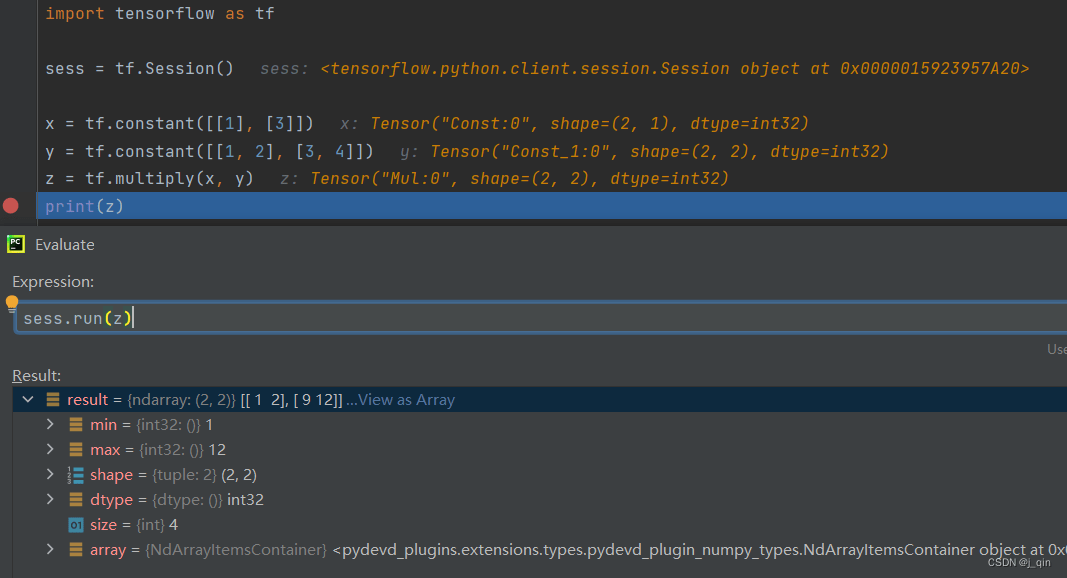Inner product (according to the understanding of the inner product operation under IPNN), the inner product of vectors, the dot multiplication operation is performed on two vectors, which is the operation of multiplying the corresponding bits of the two vectors one by one and then summing, A=[ x1,x2,x3], B=[y1,y2,y3], get [x1*y1 + x2*y2 + x3*y3],It can be seen that the result of the inner product of two vectors is a scalar. The inner product can be expressed using the following formula,【4】Summary## 【2】Gating mechanism

The gating mechanism is widely used in recommendation systems, such as the multi-task model MMOE, PLE, etc. Its main function is equivalent to a regulating valve to control the degree of information inflow or outflow. The gating mechanism is also used in the CTR ranking model. for:

(1) Learning feature importance: Feature cross combination is very important in the ranking model. Selecting useful features for cross combination can improve the efficiency of feature crossover. Therefore, the threshold mechanism can be used to dynamically learn the importance of each feature and weaken unimportant features. Strengthen the role of important features, which in turn can improve the efficiency of feature crossover and model effects;

(2) Perceived contextual information: The same word in the NLP field has different meanings in different contexts (such as “apple”, like Apple mobile phones, like apples and bananas). By perceiving contextual information, more accurate expressions can be obtained. The control mechanism takes all features as input, dynamically generates a mask containing context information, and integrates context information into features through Hadamard product, thereby improving feature expression and crossover capabilities.

(3) Learning different feature distributions: In the case of the same input, control the outflow of information through the gating network, and provide different inputs for different parallel sub-networks, so that different sub-networks can learn different feature distributions.

## 1. FiBiNET

(1) The SENET layer learns the weight of each feature field, and then multiplies the feature weight with the embedding to participate in the subsequent cross calculation, which plays a gating role, weakens unimportant features, strengthens important features, and improves the model effect.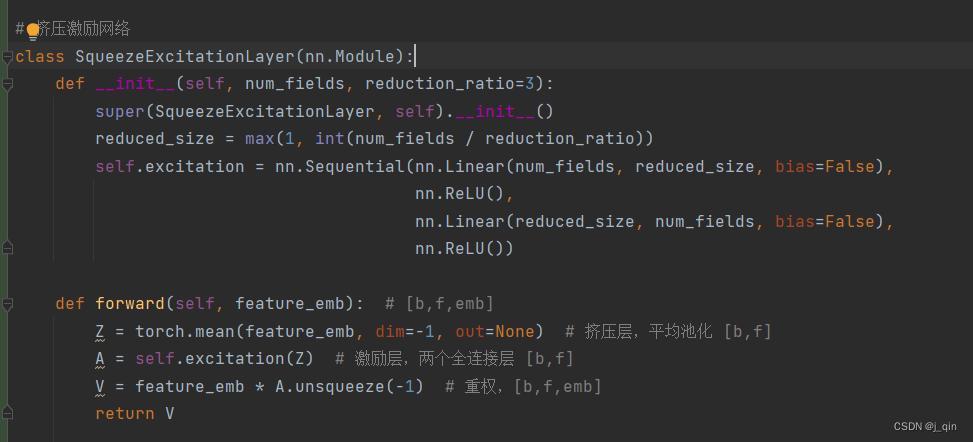## 2. GateNet

(1) Introduce the Gate mechanism in the embedding layer and the DNN hidden layer, and select important feature information to participate in feature cross combination. For a feature field. embedding layer Gate, embedding vector as input, through a layer of FC, to get the weight of the feature fields, there are two calculation methods, Vector-Wise: embedding vector through a layer of FC, the output node is 1, Get the feature field weight value, and multiply the weight value with the original embedding vector. Bit-Wise: The embedding vector passes through a layer of FC, the number of output nodes is the same as the embedding dimension, and the feature field weight vector is obtained, and the weight vector and the original embedding vector are entered into the Hadamard product.

(2) Hidden layer Gate, is similar to the above-mentioned Bit-Wise. The hidden layer features are used as input, and a weight vector with the same dimension as the input feature is obtained through a layer of FC. The weight vector and the original feature vector are Hadamard product.

## 3. EDCN

(1) Including two core modules: Bridge module: After the explicit feature crossing and implicit feature crossing are performed separately, they are fused, and the interaction between the layers of the sub-network is better captured through collaborative work Signal. Regulation module: Different features are suitable for different cross functions. Through the field-wise gating network, the fused features are separated, so that the subsequent explicit and implicit cross structure input feature distributions are different.(1) Instance-Guided Mask: It consists of two layers of FC. First, the context feature aggregation is performed, and then the dimension is mapped so that the mask dimension is the same as the input feature. The aggregation layer is wider than the projection layer because the size of the projection layer needs to be equal to the feature embedding layer. The instance-guided mask can be seen as a special bit-wise attention or gate structure. Vmask can be directly regarded as the weight of each bit, which can strengthen important features and reduce the influence of noise on the model.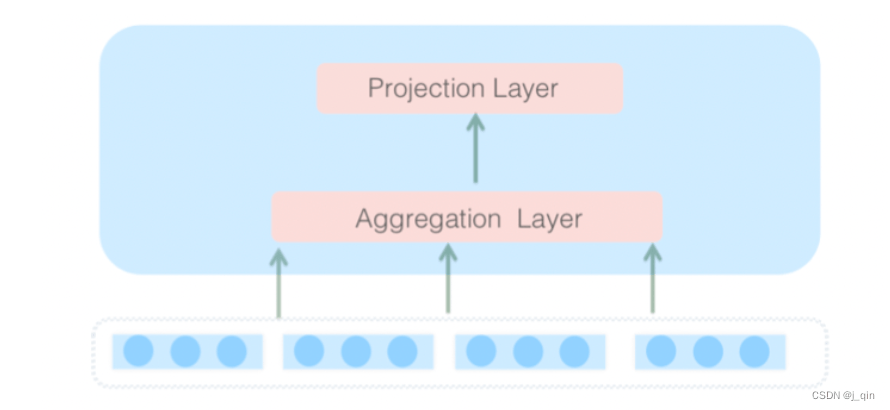(2) MaskBlock: When the embedding layer is used as input, the Instance-Guided Mask is obtained according to all input features, and then the embedding layer vector passes through the LayerNorm, and then performs Hadamard product with the mask, and then passes through FC + LayerNorm + ReLU for feature crossover.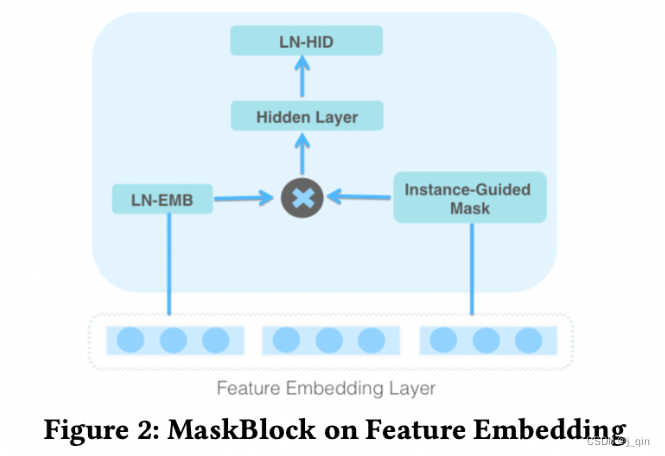```class SerialMaskNet(nn.Module):
def __init__(self, input_dim, output_dim=None, output_activation=None, hidden_units=[],
hidden_activations="ReLU", reduction_ratio=1, dropout_rates=0, layer_norm=True):
if not isinstance(dropout_rates, list):
dropout_rates = [dropout_rates] * len(hidden_units)
if not isinstance(hidden_activations, list):
hidden_activations = [hidden_activations] * len(hidden_units)
self.hidden_units = [input_dim] + hidden_units
for idx in range(len(self.hidden_units) - 1):
self.hidden_units[idx],
self.hidden_units[idx+1],
hidden_activations[idx],
reduction_ratio,
dropout_rates[idx],
layer_norm))
fc_layers = []
if output_dim is not None:
fc_layers.append(nn.Linear(self.hidden_units[-1], output_dim))
if output_activation is not None:
fc_layers.append(get_activation(output_activation))
self.fc = None
if len(fc_layers) > 0:
self.fc = nn.Sequential(*fc_layers)

def forward(self, V_emb, V_hidden):
v_out = V_hidden
for idx in range(len(self.hidden_units) - 1):
v_out = self.mask_blocks[idx](V_emb, v_out) # [b,block_dim]
if self.fc is not None:
v_out = self.fc(v_out) # [b,1]
return v_out

def __init__(self, input_dim, hidden_dim, output_dim, hidden_activation="ReLU", reduction_ratio=1,
dropout_rate=0, layer_norm=True):
self.mask_layer = nn.Sequential(nn.Linear(input_dim, int(hidden_dim * reduction_ratio)),
nn.ReLU(),
nn.Linear(int(hidden_dim * reduction_ratio), hidden_dim))
hidden_layers = [nn.Linear(hidden_dim, output_dim, bias=False)]
if layer_norm:
hidden_layers.append(nn.LayerNorm(output_dim)) # normalization
hidden_layers.append(get_activation(hidden_activation))
if dropout_rate > 0:
hidden_layers.append(nn.Dropout(p=dropout_rate))
self.hidden_layer = nn.Sequential(*hidden_layers)

def forward(self, V_emb, V_hidden):
return v_out```

```class ParallelMaskNet(nn.Module):
def __init__(self, input_dim, output_dim=None, output_activation=None, num_blocks=1, block_dim=64,
hidden_units=[], hidden_activations="ReLU", reduction_ratio=1, dropout_rates=0,
layer_norm=True):
self.num_blocks = num_blocks
input_dim,
block_dim,
hidden_activations,
reduction_ratio,
dropout_rates,
layer_norm) for _ in range(num_blocks)])

self.dnn = MLP_Layer(input_dim=block_dim * num_blocks,
output_dim = output_dim,
hidden_units=hidden_units,
hidden_activations=hidden_activations,
output_activation=output_activation,
dropout_rates=dropout_rates)

def forward(self, V_emb, V_hidden): # [b,f*emb]
block_out = []
for i in range(self. num_blocks):
block_out.append(self.mask_blocks[i](V_emb, V_hidden)) # num_blocks [b, block_dim]
concat_out = torch.cat(block_out, dim=-1) # [b,num_blocks*block_dim]
v_out = self.dnn(concat_out) # feed-forward layer [b,1]
return v_out

def __init__(self, input_dim, hidden_dim, output_dim, hidden_activation="ReLU", reduction_ratio=1,
dropout_rate=0, layer_norm=True):
self.mask_layer = nn.Sequential(nn.Linear(input_dim, int(hidden_dim * reduction_ratio)),
nn.ReLU(),
nn.Linear(int(hidden_dim * reduction_ratio), hidden_dim))
hidden_layers = [nn.Linear(hidden_dim, output_dim, bias=False)]
if layer_norm:
hidden_layers.append(nn.LayerNorm(output_dim)) # normalization
hidden_layers.append(get_activation(hidden_activation))
if dropout_rate > 0:
hidden_layers.append(nn.Dropout(p=dropout_rate))
self.hidden_layer = nn.Sequential(*hidden_layers)

def forward(self, V_emb, V_hidden):